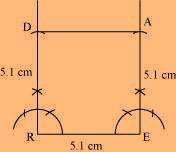4.3    SOME SPECIAL CASES:

Some Special Cases:

There are some special cases in which we can construct the quadrilateral with less number of measurements also.

Draw the following:

1. A square READ with RE =  5.1 cm.

Solution: Given:  RE   =   5.1 cm.

Steps of construction:

(i)   Draw RE   =   5.1 cm.

(ii)   At point E, construct an angle of  90  and draw an arc of radius 5.1 cm, which intersects at  point A.

(iii)   At point R, draw an arc of radius 5.1 cm at point A, draw another arc of radius 5.1 cm which intersects the first arc at point D.1. A rhombus whose diagonals are 5.2 cm and 6.4 cm.

Given:  Diagonals of a rhombus  are   AC  =  5.2 cm  and    BD  = 6.4 cm.

To construct: A rhombus ABCD.

Steps of construction:

(c)   Draw two arcs on both sides of AC of radius 3.2 cm from intersection point O, which intersects at B and D.

(d)   Join AB, BC, CD and DA.

The  required rhombus is  ABCD.

1. A rectangle with adjacent sides of  length 5 cm  and  4  cm.

Given:  MN   =  5 cm and MP   =   4 cm.

To construct: A rectangle MNOP

Steps of construction:

(a)   Draw a segment MN   =   5 cm.

(b)   At points M and N, draw perpendiculars of lengths 4 cm and produce them.

(c)   Taking centres M and N, draw two arcs of 4 cm each, which intersect P and Q respectively.

(d)   Join side PO.

The required rectangle  is   MNOP.

1.  A parallelogram OKAY  where    OK   = 5.5 cm  and  KA  =  4.2 cm.

Given:  OK   =   5.5 cm  and  KA   =   4.2 cm.

To construct: A parallelogram  OKAY.

Steps of construction:

(a)   Draw a line segment OK   =   5.5 cm.

(b)   Draw an angle of  90  at K and draw an arc of radius KA   =   4.2 cm,

which intersects at point A.

(c)   Draw another arc of radius AY  = 5.5 cm  and at point O, draw another arc of radius 4.2 cm

which intersects at Y.

(d)   Join AY and OY.

The required parallelogram  is  OKAY.

(a)   Draw AC   =   5.2 cm and draw perpendicular bisectors on AC.

(b)   Since, diagonals bisect at mid-point O, therefore get half of 6.4 cm, i.e., 3.2 cm.